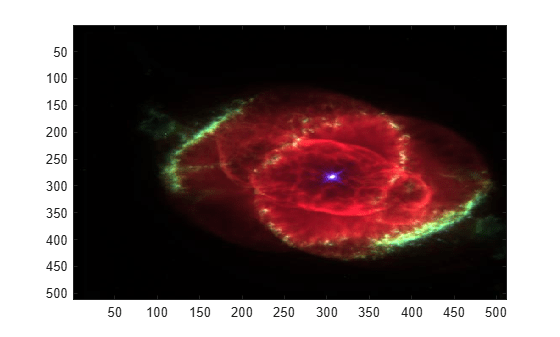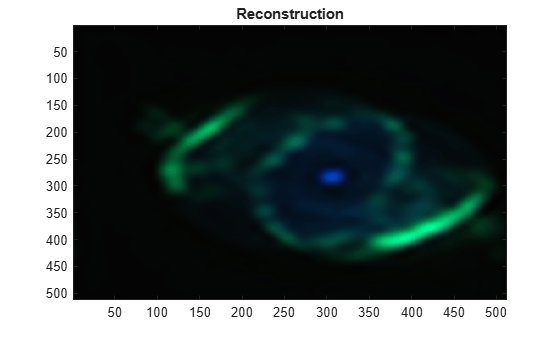# iswt2

Inverse discrete stationary 2-D wavelet transform

## Syntax

``X = iswt2(swc,wname)``
``X = iswt2(swc,LoR,HiR)``
``X = iswt2(A,H,V,D,wname)``
``X = iswt2(A,H,V,D,LoR,HiR)``

## Description

````X = iswt2(swc,wname)` returns the inverse discrete stationary 2-D wavelet transform of the wavelet decomposition `swc` using the wavelet `wname`. The decomposition `swc` is the output of `swt2`. Note`swt2` uses double-precision arithmetic internally and returns double-precision coefficient matrices. `swt2` warns if there is a loss of precision when converting to double. ```
````X = iswt2(swc,LoR,HiR)` uses the specified lowpass and highpass wavelet reconstruction filters `LoR` and `HiR`, respectively.```

example

````X = iswt2(A,H,V,D,wname)` uses the approximation coefficients array `A` and detail coefficient arrays `H`, `V`, and `D`. The arrays `H`, `V`, and `D` contain the horizontal, vertical, and diagonal detail coefficients, respectively. The arrays are the output of `swt2`.If the decomposition `swc` or the coefficient arrays `A`, `H`, `V`, and `D` were generated from a multilevel decomposition of a 2-D matrix, the syntax ```X = iswt2(A(:,:,end),H,V,D,wname)``` reconstructs the 2-D matrix.If the decomposition `swc` or the coefficient arrays `A`, `H`, `V`, and `D` were generated from a single-level decomposition of a 3-D array, the syntax ```X = iswt2(A(:,:,1,:),H,V,D,wname)``` reconstructs the 3-D array.```

example

````X = iswt2(A,H,V,D,LoR,HiR)` uses the lowpass and highpass wavelet reconstruction filters `LoR` and `HiR`, respectively.If the decomposition `swc` or the coefficient arrays `A`, `H`, `V`, and `D` were generated from a multilevel decomposition of a 2-D matrix, the syntax ```X = iswt2(A(:,:,end),H,V,D,LoR,HiR)``` reconstructs the 2-D matrix.If the decomposition `swc` or the coefficient arrays `A`, `H`, `V`, and `D` were generated from a single-level decomposition of a 3-D array, the syntax ```X = iswt2(A(:,:,1,:),H,V,D,LoR,HiR)``` reconstructs the 3-D array.```

## Examples

collapse all

Show perfect reconstruction using `swt2` and `iswt2` with an orthogonal wavelet.

```load woman [Lo_D,Hi_D,Lo_R,Hi_R] = wfilters('db6'); [ca,chd,cvd,cdd] = swt2(X,3,Lo_D,Hi_D); recon = iswt2(ca,chd,cvd,cdd,Lo_R,Hi_R); norm(X-recon)```
```ans = 1.0126e-08 ```

This example shows how to reconstruct an RGB image from a multilevel stationary wavelet decomposition using approximation and detail coefficient arrays.

Load an RGB image. An RGB image is also referred to as a truecolor image. The image is a 3-D array of type `uint8`. Since `swt2` requires that the first and second dimensions both be divisible by a power of 2, extract a portion of the image and view it.

```imdata = imread('ngc6543a.jpg'); x = imdata(1:512,1:512,:); image(x)```Obtain the level 4 stationary wavelet decomposition of the image using the `db4` wavelet. Return the approximation coefficients and horizontal, vertical, and detail coefficients as separate arrays.

`[a,h,v,d] = swt2(x,4,'db4');`

Reconstruct an image using the green and blue components of the approximation coefficients. Display the reconstruction.

```a2 = zeros(size(a)); a2(:,:,2:3,4)=a(:,:,2:3,4); xrec = iswt2(a2,0*h,0*v,0*d,'db4'); xrec2 = (xrec-min(xrec(:)))/(max(xrec(:))-min(xrec(:))); image(xrec2) title('Reconstruction')```## Input Arguments

collapse all

Stationary wavelet decomposition, specified as a 3-D or 4-D array. The decomposition contains the approximation and detail coefficients of the 2-D stationary wavelet transform (SWT). The stationary wavelet decomposition is the output of `swt2`.

Data Types: `double`

Analyzing wavelet, specified as a character vector or string scalar. `iswt2` supports only Type 1 (orthogonal) or Type 2 (biorthogonal) wavelets. See `wfilters` for a list of orthogonal and biorthogonal wavelets. The specified wavelet must be the same wavelet used to obtain the approximation and detail coefficients.

Wavelet reconstruction filters, specified as a pair of even-length real-valued vectors. `LoR` is the lowpass reconstruction filter, and `HiR` is the highpass reconstruction filter. The lengths of `LoR` and `HiR` must be equal. See `wfilters` for additional information.

Approximation coefficients, specified as a multidimensional array. The array is the output of `swt2`.

Data Types: `double`

Detail coefficients, specified as multidimensional arrays of equal size. `H`, `V`, and `D` contain the horizontal, vertical, and diagonal detail coefficients, respectively. The arrays are the output of `swt2`.

Data Types: `double`

## Output Arguments

collapse all

Reconstruction, returned as a 2-D matrix or 3-D array.

If `swc` or (`A`,`H,V,D`) are obtained from an indexed image analysis or a truecolor (RGB) image analysis, then `X` is an m-by-n matrix or an m-by-n-by-3 array, respectively.

## Compatibility Considerations

expand all

Behavior changed in R2017b

 Nason, G. P., and B. W. Silverman. “The Stationary Wavelet Transform and Some Statistical Applications.” In Wavelets and Statistics, edited by Anestis Antoniadis and Georges Oppenheim, 103:281–99. New York, NY: Springer New York, 1995. https://doi.org/10.1007/978-1-4612-2544-7_17.

 Coifman, R. R., and D. L. Donoho. “Translation-Invariant De-Noising.” In Wavelets and Statistics, edited by Anestis Antoniadis and Georges Oppenheim, 103:125–50. New York, NY: Springer New York, 1995. https://doi.org/10.1007/978-1-4612-2544-7_9.

 Pesquet, J.-C., H. Krim, and H. Carfantan. “Time-Invariant Orthonormal Wavelet Representations.” IEEE Transactions on Signal Processing 44, no. 8 (August 1996): 1964–70. https://doi.org/10.1109/78.533717.

## Support#### Try MATLAB, Simulink, and Other Products

Get trial now